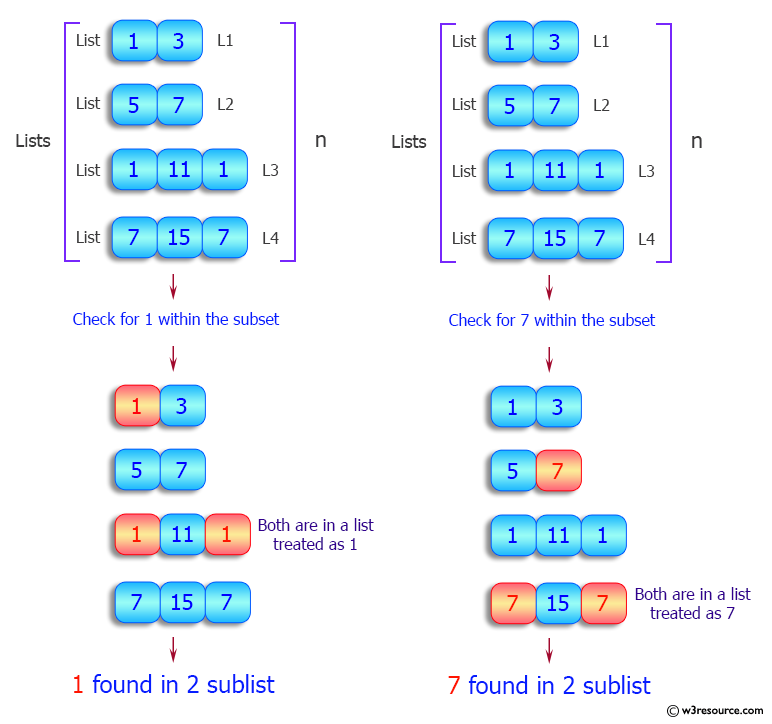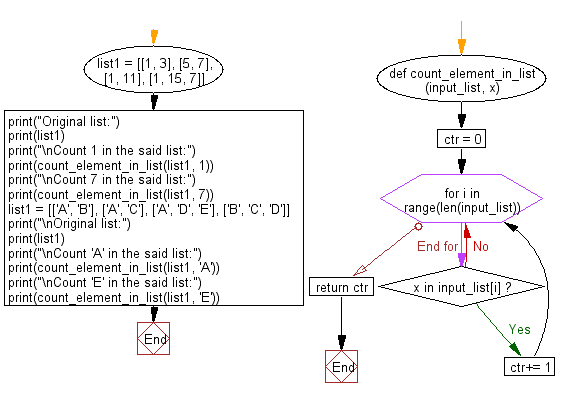﻿ Python: Count the number of sublists contain a particular element - w3resource# Python: Count the number of sublists contain a particular element

## Python List: Exercise - 93 with Solution

Write a Python program to count the number of sublists contain a particular element.

Sample Solution:

Python Code:

``````def count_element_in_list(input_list, x):
ctr = 0
for i in range(len(input_list)):
if x in input_list[i]:
ctr+= 1

return ctr

list1 = [[1, 3], [5, 7], [1, 11], [1, 15, 7]]
print("Original list:")
print(list1)
print("\nCount 1 in the said list:")
print(count_element_in_list(list1, 1))
print("\nCount 7 in the said list:")
print(count_element_in_list(list1, 7))

list1 = [['A', 'B'], ['A', 'C'], ['A', 'D', 'E'], ['B', 'C', 'D']]
print("\nOriginal list:")
print(list1)
print("\nCount 'A' in the said list:")
print(count_element_in_list(list1, 'A'))
print("\nCount 'E' in the said list:")
print(count_element_in_list(list1, 'E'))
```
```

Sample Output:

```Original list:
[[1, 3], [5, 7], [1, 11], [1, 15, 7]]

Count 1 in the said list:
3

Count 7 in the said list:
2

Original list:
[['A', 'B'], ['A', 'C'], ['A', 'D', 'E'], ['B', 'C', 'D']]

Count 'A' in the said list:
3

Count 'E' in the said list:
1
```

Pictorial Presentation:Flowchart:## Visualize Python code execution:

The following tool visualize what the computer is doing step-by-step as it executes the said program:

Python Code Editor:

Have another way to solve this solution? Contribute your code (and comments) through Disqus.

What is the difficulty level of this exercise?

Test your Python skills with w3resource's quiz

﻿

## Python: Tips of the Day

```print(2_000_000)
```2000000